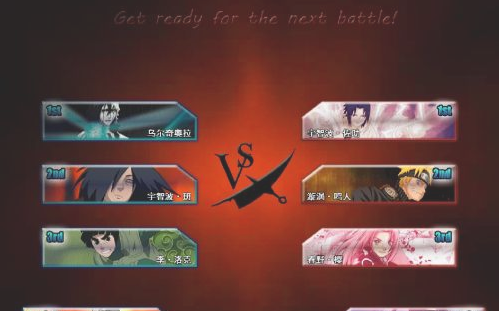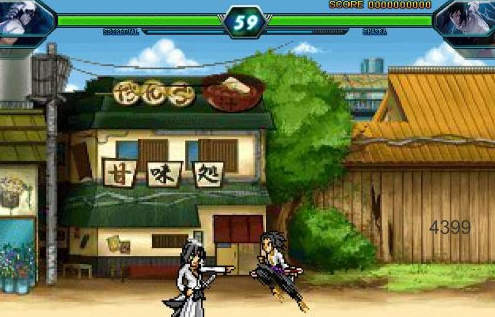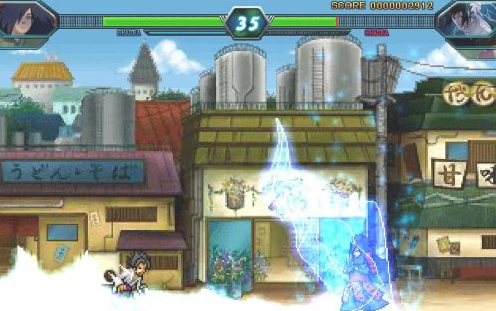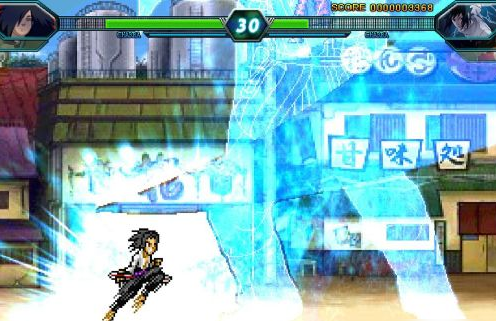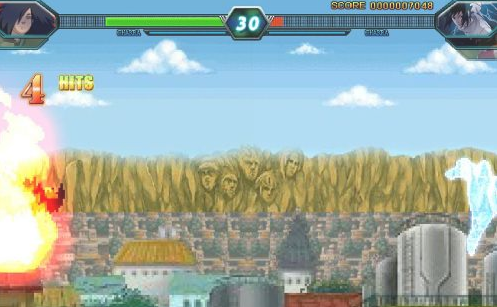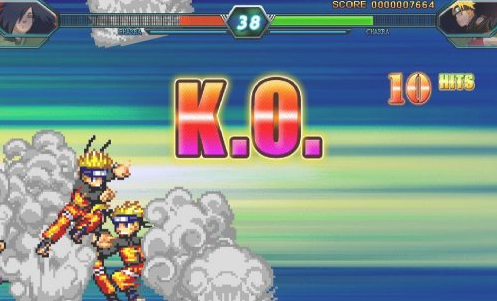• 游戏
• 软件
• 专题## 游戏介绍

### 死神vs火影暗殿改版本说明：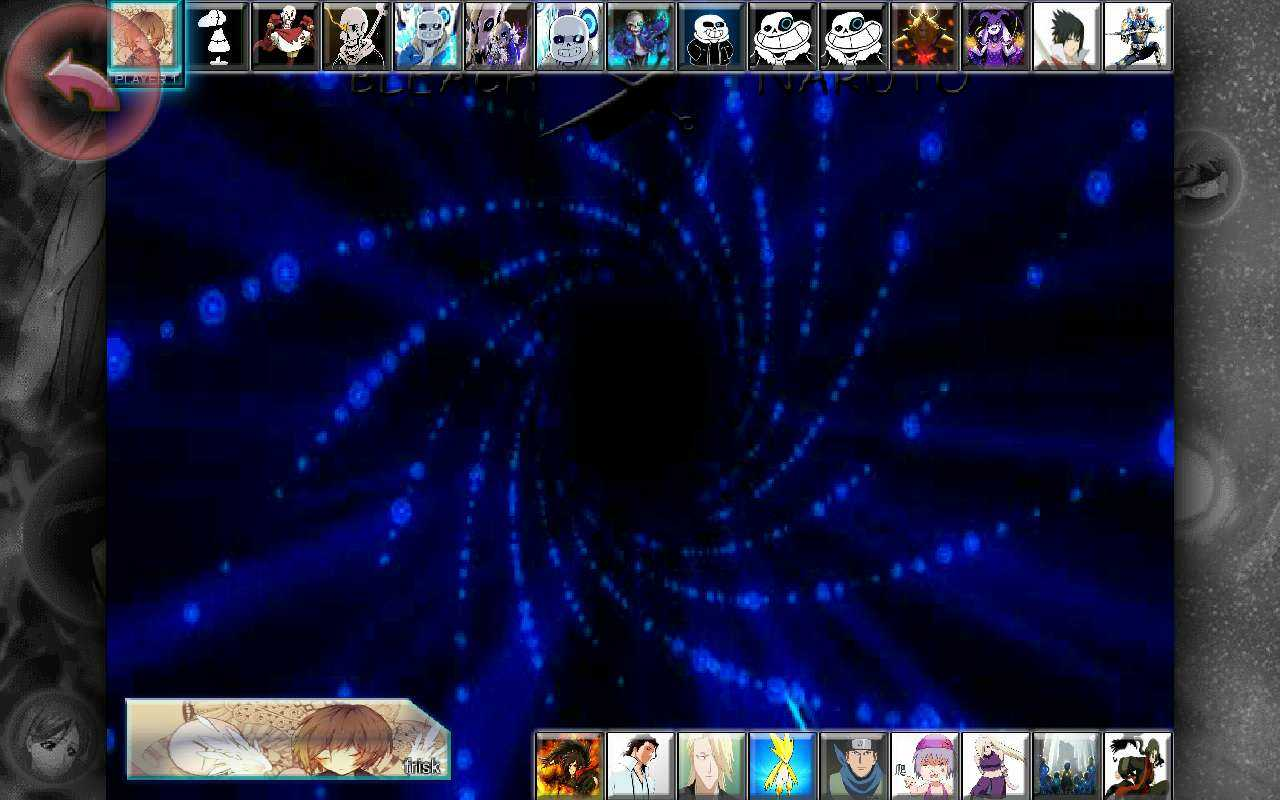### 死神vs火影出招表：

①格式采用大写字母，键盘为默认键;招式间采用“+”作为连接;连招末端若能接多种技能，采用“/”隔开，这样更简洁，不啰嗦重复。

②展示的幽连遵循{原始起手，一次幽步，大招收尾}的原则，吧友可在吸收理解后自行调整。

②展示的以实用幽连 —— 稳定，高伤的幽连为主，不讨论无大作用的幽连(如J+SL+I)。

③幽连中尽量但不一定不重复招式，暂且不讨论辅助连、地形连，断连，但可能会穿插版边连。

④每个人物展示一个或多个幽连，难度有低有高，实用度也一样。

①朽木白哉

KJ+JJ+SJ+WJ+SL+KJ+JJ+SJ+WJ+WU+I

KJ可加可不加，看技术;WJ注意只要打第一下

③阿散井·恋次

U+WU+SL+KJU+JJJ+WJ+WI

JJJ+WJ+WL+KJU+JJJ+WJ+WI

U+WU+KJU+JJJ+SU+WL+KJU+JJJ+WJ+L+I

⑤黑崎一护——始解

KJ+JJ+SU+SL+JJJ+SU+I

KJ+JJ+WJ+WL+KJ+JJJ+SU+I

WJ与KJ间的衔接要注意

KJ+JJJ+SU+SL+小跑+KJ+JJJ+SU+I

⑥漩涡鸣人

KJU+JJJ+WJ+WU+WL+KJU+JJ+U+I

⑦春野樱

WJ+KJU+JJJ+SJ+SL+JJ+WJ+KJU+JJJ+I

WJ起手的幽连

KU+JJ+WJ+KJ+JJJ+SU+SL+KJU+JJ+WJ+KI

KU起手的幽连

KJU+JJJ+SU+KI

⑧宇智波·佐助

KJ+WU+KU+JJJ+SL+KJ+WU+KU+JJJ+I

KJU+JJ+WJ+SL+KJU+JJJ+I

SU+WU+WL+KU+JJJ+I

⑨旗木·卡卡西

U+SU+WL+KJ+JJ+I

JJ+KJ+J+U+SL+KJ+JJJ+WI

10、李·洛克

U+KJ+JJ+SJ+SL+KJ+JJJ+U+KJ+WU+U+I

11、茶渡·泰虎

U+JJJ+SU+SL+JJJ+SU+WI

U+KJ+JJJ+SJ+SL+U+JJJ+SU+WI

12、日番谷·冬狮郎 - 卍解

U+JJJ+WL+KJ+JJ+WJ+WI

13、石田雨龙

KJ+JJJJ+SL+KJ+JJJJ+I

14朽木·露琪亚 — 始解

KJ+JJJJ+SL+KJ+JJJ+SJ+WI

SU+KJ+JJJ+SJ+SL+SU+JJJ+SJ+WJ+KJ+I

15、黑崎一护——卍解

U+JJJ+WL+KJ+J+I

SJ+WJ+KJ+JJJ+WL+KJ+JJJ+WI

16、漩涡鸣人——一尾

KJU+U+JJJJ+WL+KJU+JJJJ+WI

17、手鞠

WJ+SL+KJ+JJJ+WI

18、宇智波佐助——咒印化

KJ+SJSJ+JJJ+WJ+SL+KJ+SJSJ+JJJ+WJ+WI

SU+JJJ+KJ+SL+KJ+SJSJ+JJJ+KJ+I

19、日向宁次

WJ+JJJ+KJU+SJJ+U+SL+JJJ+KJU+SJ+I

KJU+JJJJ+SL+KJU+JJJ+L+WI

20、宇智波·鼬

U+KJ+JJ+SL+U+KJ+JJ+I

U+KJ+JJJ+KJ+WU+SL+U+KJ+JJJ+WI

KJ与WU的衔接较难，慎用

21、乌尔奇奥拉

SU+WJ+WL+KJ+JJJ+I

KJ+JJ+WJ+WL+KJ+JJJ+I

KJ+JJJ+SJ+SL+KJ+JJ+WJ+WI

22、市丸银

KJ+U+JJJJ+SJ+SL+KJ+U+JJJJ+SJ+I

23、我爱罗

KJ+JJJ+U+SL+KJ+JJ+WJ+SJ+WI

KJ+JJ+WJ+SJ+SL+KJ+JJJ+U+I

24、佩恩

JJ+SJ+WJ+KJ+SL+JJ+SJ+WJ+WI

SJ+JJJ+WJ+KJ+SL+SJ+JJJ+WJ+KJI

25、小南

KJ+JJJ+SU+SL+KJ+JJJ+I

KJ+JJ+SJ+WJ+KJ+SL+KJ+JJ+SJ+WJ+KJI

28、黑崎一护·零段

KJ+JJJ+SJ+SU+SL+KJ+JJJ+SJ+SU+WI

PS：零段的I可以用在幽步中

30、宇智波·带土

(近身按住方向键)KJ+JJ+WJJ+SJ+SL+JJ+WJJJ+I

(近身按住方向键)KJ+JJ+SJ+小跑+WU+SL+JJ+WJJJ+I

31、宇智波佐助——须佐能乎

JJJJ+SJJ+SL+JJJJ+SJ+WJ+WI

JJJJ+SJ+WJ+KJ+SL+WJ+KI

32、漩涡鸣人——仙人模式

U+KJ+JJJJ+SU+SL+KJ+JJJJ+SU+I

KJ+JJJJ+SU+U+WJ+SL+SJJJ+WU+KI

SJJJ+KJ+JJ+SU+U+WJ+SL+SJJ+U+WJ+WI

PS：从以上几个GIF中可以看出仙鸣的纽带性非常好，但是为了效果都采用了伤害最大化连招，追求稳定的话可以用万金油JJJ+U+WJ

33、绯村·剑心

JJ+SJJ+SL+SJ+JJ+I

SJJ+KJ+JJ+I+SL+JJ+SJJ+WU+KJU

200%血，不以大招收尾，对技术有一定要求的连招

34、黑崎一护——虚化

U+JJJJ+SL+U+JJJJ+SU+I

KJ+U+JJJJ+SU+WL+KJ+U+JJJJ+SU+I

KJ+JJJJJ+L+WJ+WL+KJ+JJJJJ+L+WI

35、漩涡鸣人——四尾模式

KJU+JJJJ+WL+KJU+JJ+I

PS：JJJJ+WI的连招会断，所以在实战中斟酌使用

36、乌尔奇奥拉——归刃

KJ+JJJJ+SL+KJ+JJJ+SJ+WJ+KI

37、黑崎一护——白虚

KJ+JJJJ+WL+KJ+JJJJ+WI

KJ+JJ+WJ+WU+WL+KJ+JJJJ+WI

2.3新增加人物篇

30、斑目一角

KJ+JJ+WJ+SJ+WL+KJ+JJ+WJJ+WI

KJ+JJJ+WJ+KJU+SL+KJ+JJJ+WJ+WI

41、迪达拉

JJ+WJ+SJ+KJ+SL+JJ+WJ+SJ+WI

42、朽木白哉——卍解

WJ+J+SL+JJ+WJ+WI

2.5更新人物篇

43、自来也

KJ+JJJ+SUJ+SL+KJ+JJJ+WJ+WI

KJ+JJ+WJ+SJ+WU+WL+KJ+JJJ+SU+L+WI

45、带土——阿飞

U+JJJ+SJ+SL+U+JJJ+SJ+WI

47、漩涡香磷

SJ+WJ+WL+KJ+JJJ+WJ+I

KJ+JJJ+U+SL+KJ+JJJ+SU+I

2.5更新人物篇

46、天枰·重吾

KJ+JJ+SJ+U+SL+L+KJ+JJ+SJ+I

### 死神vs火影怎么抓取破防?